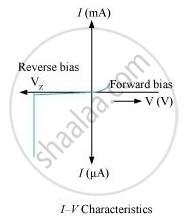Share

# How is a Zener Diode Fabricated So as to Make It a Special Purpose Diode? Draw I-v Characteristics of Zener Diode and Explain the Significance of Breakdown Voltage.Explain Briefly, with the Help of a - Physics

#### Question

How is a zener diode fabricated so as to make it a special purpose diode? Draw I-V characteristics of zener diode and explain the significance of breakdown voltage.

Explain briefly, with the help of a circuit diagram, how a p-n junction diode works as a half wave rectifier.

#### Solution

Zener is fabricated such that both the p-type and the n-type are highly doped. This makes the depletion region thin. When an electric field is applied, a high electric field appears across the thin depletion region. When the electric field becomes very high, it knocks off electrons from the host atoms to create a large number of electrons. This results in a large value of current inside the circuit.Zener has a sharp breakdown voltage and this property of zener is used for voltage regulation.

An ac current has a positive half cycle and a negative half cycle. A pn junction allows current to pass only in one direction and that is when it is forward biased.

When a positive half-cycle occurs, the p-side has a lower potential. Therefore, the diode is now forward biased and therefore conducts and this positive cycle is available for the load.

When a negative half cycle occurs, the n-side has a higher potential than the p-side. Hence, the diode is now reverse biased and thus, does not conduct. As a result, this positive half cycle also does not conduct. Therefore, it does not appear at the load and is cut-off.

We obtain a waveform, which has only positive half cycles and therefore it is called half-wave rectifier.

Is there an error in this question or solution?